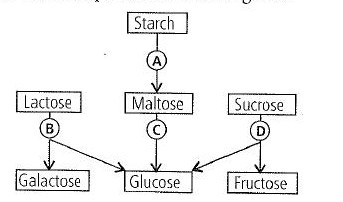# NEET Zoology Digestion and Absorption Questions Solved

The following is a scheme showing the fate of carbohydrates during digestion in the human alimentary canal. Identify the enzymes acting at stages indicated as A, B, C and D. Choose the correct option from those given.(a) A = Amylase, B = Maltase, C = Lactase,D = Invertase

(b) A = Amylase, B = Maltase, C = Invertase,D = Lactase

(c) A = Amylase, B = Invertase, C = Maltase, D = Lactase

(d) A = Amylase, B = Lactase, C = Maltase, D = Invertase